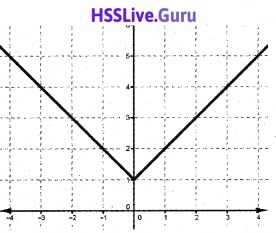# Plus One Maths Chapter Wise Questions and Answers Chapter 2 Relations and Functions

Students can Download Chapter 2 Relations and Functions Questions and Answers, Plus One Maths Chapter Wise Questions and Answers helps you to revise the complete Kerala State Syllabus and score more marks in your examinations.

## Kerala Plus One Maths Chapter Wise Questions and Answers Chapter 2 Relations and Functions

### Plus One Maths Relations and Functions Three Mark Questions and Answers

Plus One Maths Relations And Functions Previous Questions And Answers Question 1.
Let A = {1, 2, 3, 4, 6}. Let R be the relation on A defined by R = {(a, b): a, b ∈ A, b is exactly divisible by a}

1. Write R in roster form. (1)
2. Find the domain of R. (1)
3. Find the range of R. (1)

1. R = {(1, 1), (1, 2), (1, 3), (1, 4), (1, 6), (2, 4), (2, 2), (4,4), (6,6), (3,3), (3,6)}
2. Domain of R = {1, 2, 3, 4, 6}
3. Range of R = {1, 2, 3, 4, 6}

Plus One Maths Chapter Wise Questions And Answers Pdf Question 2.
Determine the domain and range of the relation R defined by R = {(x, x + 5) : x ∈ {0, 1, 2, 3, 4, 5}}
R = {(0, 5), (1, 6), (2, 7), (3, 8), (4, 9),(5, 10)}
Domain of R = {0, 1, 2, 3, 4, 5}
Range of R = {5, 6, 7, 8, 9, 10}

Relations And Functions Class 11 Important Questions Pdf Question 3.
A function f is defined as f(x) = 2x – 5, Write down the values of f(0), f(7), f(-3).
Given; f(x) = 2x – 5
f(0) = -5;
f(7) = 2(7) – 5 = 14 – 5 = 9
f(-3) = 2(-3) – 5 = -6 – 5 = -11

Hsslive Maths Textbook Answers Plus One Question 4.
Find the range of the following functions.

1. f(x) = 2 – 3x, x ∈ R, x>0 (1)
2. f(x) = x2 + 2, x is a real number. (1)
3. f(x) = x, x is a real number. (1)

1. Given; f(x) = 2 – 3x is a first degree polynomial function, therefore the range is R.
2. Given; f(x) = x2 + 2, The range of x2 is [0, ∞) , then the range of f(x) = x2 + 2 is [2, ∞)
3. Given; f(x) = x is the identity function, therefore the range is R.

### Plus One Maths Relations and Functions Four Mark Questions and Answers

Plus One Maths Chapter Wise Questions And Answers Question 1.
Let A = {1, 2}, B = {1, 2, 3, 4}, C = {5, 6} and D = {5, 6, 7, 8}. Verify that

1. A × (B ∩ C) = (A × B) ∩ (A × C) (2)
2. A × C is a subset of B × D (2)

1. A × (B ∩ C) ={1, 2} × Φ = Φ
A × B = {(1, 1), (1, 2), (1, 3), (1, 4), (2, 1), (2, 2), (2, 3), (2, 4)}
A × C = {(1, 5), (1, 6), (2, 5), (2, 6)}
(A × B) ∩ (A × C) = Φ
Hence; A × (B ∩ C) = (A × B) ∩ (A × C)

2. A × C = {(1, 5), (1, 6), (2, 5), (2, 6)}
B × D = {1, 2, 3, 4} × {5, 6, 7, 8}
= {(1, 5), (1, 6), (1, 7), (1, 8), (2, 5), (2, 6), (2, 7), (2, 8), (3, 5), (3,6), (3, 7), (3, 8), (4, 5), (4, 6), (4, 7), (4, 8)}
Hence A × C is a subset of B × D.

Relations And Functions Class 11 Important Questions Question 2.
The arrow diagram given below shows a relation R from P to Q. Write the relation in roster form, set-builder form. Find its domain and range.R – {(9, -3), (9, 3), (4, -2), (4, 2), (25, -5), (25, 5)}
R = {{x, y) : y2 = x}
Domain of R = {9, 4, 25}
Range of R = {5, 3, 2, -2, -3, -5}

Question 3.
Find the domain of the following.

1. f(x) = $$\frac{x^{2}+2 x+1}{x^{2}-8 x+12}$$ (2)
2. f(x) = $$\frac{x^{2}+3 x+5}{x^{2}-5 x+4}$$ (2)

1. Given; f(x) = $$\frac{x^{2}+2 x+1}{x^{2}-8 x+12}$$
The function is not defined at points where the denominator becomes zero.
x2 – 8x +12 = 0 ⇒ (x – 6)(x – 2) = 0 ⇒ x = 2, 6
Therefore domain of fis R – {2, 6}.

2. Given; f(x) = $$\frac{x^{2}+3 x+5}{x^{2}-5 x+4}$$
The function is not defined at points where the denominator becomes zero.
x2 – 5x + 4 = 0 ⇒ (x – 4)(x -1) = 0 ⇒ x = 1, 4
Therefore domain of f is R – {1, 4}.

Plus One Maths Questions And Answers Question 4.
Let f(x) = $$=\sqrt{x}$$ and g(x) = x be two functions defined over the set of nonnegative real numbers. Find (f + g)(x), (f – g)(x), (fg)(x) and $$\left(\frac{f}{g}\right)(x)$$.
(f + g)(x) = f(x) + g(x) = $$=\sqrt{x}$$ + x
(f – g)(x) = f(x) – g(x) = $$=\sqrt{x}$$ – x
(fg)(x) = f(x) × g(x) = $$=\sqrt{x}$$ × x = $$x^{\frac{3}{2}}$$Plus One Maths Relations And Functions Question 5.
Let f(x) = x2 and g(x) = 2x + 1 be two functions defined over the set of nonnegative real numbers. Find (f + g)(x), (f – g)(x), (fg)(x) and $$\left(\frac{f}{g}\right)(x)$$.
(f + g)(x) = f(x) + g(x) = x2 + 2x + 1
(f – g)(x) = f(x) – g(x) = x2 – 2x – 1
f(fg)(x) = f(x) × g(x)
= x2(2x +1) = 2x3 + x2Relations And Functions Questions And Answers Pdf Question 6.
A = {1, 2}, B = {3, 4}

1. Write A × B
2. Write relation from A to B in roster form. (1)
3. Represent all possible functions from A to B (Arrow diagram may be used) (2)

1. A × B = {(1, 3), (1, 4), (2, 3), (2, 4)}

2. Any Subset of A × B (say R={(1, 3),(2, 4)})

3.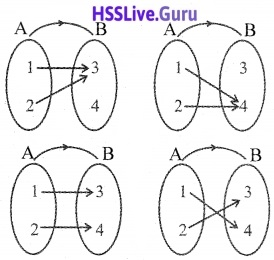### Plus One Maths Relations and Functions Six Mark Questions and Answers

Relations And Functions Class 11 Important Questions With Solutions Question 1.
Let A = {1, 2, 3}, B = {3, 4} and C = {4, 5, 6}. Find

1. A × (B ∩ C) (1)
2. (A × B) ∩ (A × C) (2)
3. A × (B ∪ C) (1)
4. (A × B) ∪ (A × C) (2)

1. A × (B ∩ C) = {1, 2, 3} × {4}
= {(1, 4), (2, 4), (3, 4)}

2. (A × B) ∩ (A × C)
= {(1, 3), (1, 4), (2, 3), (2, 4), (3, 3), (3, 4)} ∩ {(1, 4), (1, 5), (1, 6), (2, 4), (2, 5) , (2, 6), (3, 4), (3, 5), (3, 6)}
= {(1, 4), (2, 4), (3, 4)}

3. A × (B ∪ C) = {1, 2, 3} × {3, 4, 5, 6}
= {(1, 3), (1, 4), (1, 5), (1, 6), (2, 3), (2, 4), (2, 5), (2, 6), (3, 3), (3, 4), (3, 5), (3, 6)}

4. (A × B) ∪ (A × C)
= {(1, 3), (1, 4), (2, 3), (2, 4), (3, 3), (3, 4)} ∪ {(1, 4), (1, 5), (1, 6), (2, 4), (2, 5), (2, 6), (3, 4), (3, 5), (3, 6)}
= {(1, 3), (1, 4), (1, 5), (1, 6), (2, 3), (2, 4), (2, 5), (2, 6), (3, 3), (3, 4), (3, 5), (3, 6)}

Question 2.
Find the domain and range of the followingi) Given; f(x) = -|x|
D(f) = R, R(f) = (-∞, 0]

ii) Given; f(x) = $$\sqrt{9-x^{2}}$$
x can take values where 9 – x2 > 0
⇒ x2 ≤ 9 ⇒ -3 ≤ x ≤ 3 ⇒ x ∈ [-3, 3]
Therefore domain of f is [-3, 3]
Put $$\sqrt{9-x^{2}}$$ = y, where y ≥ 0
⇒ 9 – x2 = y2⇒ x2 = 9 – y2
⇒ x = $$\sqrt{9-x^{2}}$$
⇒ 9 – y2 ≥ 0 ⇒ y2 ≤ 9 ⇒ -3 ≤ y ≤ 3
Therefore range of fis [0, 3].

iii) Given; f(x) = |x – 1|
Domain of f is R
The range of |x| is [0, ∞) , then the range of
f(x) = |x -1| is [0, ∞)

iv) Given; f(x) = $$\sqrt{x-1}$$
x can take values where x – 1 ≥ 0
⇒ x ≥ 1 ⇒ x ∈ [1, ∞]
Therefore domain of fis [1, ∞]
The range of $$\sqrt{x}$$ is [0, ∞), then the range of
f(x) = $$\sqrt{x-1}$$ is [0, ∞).

### Plus One Maths Relations and Functions Practice Problems Questions and Answers

Question 1.
If (x + 1, y – 2) = (3, 1), find the values of x and y.
(x + 1, y – 2) = (3, 1) ⇒ x + 1 = 3, y – 2 = 1 ⇒ x = 2, y = 3.

Question 2.
If $$\left(\frac{x}{3}+1, y-\frac{2}{3}\right)=\left(\frac{5}{3}, \frac{1}{3}\right)$$, find the values of x and y.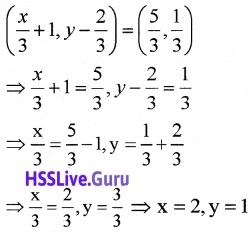Question 3.
If G = {7, 8}; H = {2, 4, 5}, find G × H and H × G.

• G × H ={(7, 2), (7, 4), (7, 5), (8, 2), (8, 4), (8, 5)}
• H × G ={(2, 7), (2, 8), (4, 7), (4, 8), (5, 7), (5, 8)}

Question 4.
if A = {-1, 1} find A × A × A
A × A ={-1, 1} × {-1, 1}
= {(-1, -1), (-1, 1), (1, -1), (1, -1)}
A × A × A
= {(-1, -1), (-1, -1), (1,-1), (1, -1)} × {-1, 1}
= {(-1, -1, -1), (-1, 1, -1), (1, -1, -1), (-1, 1, -1), (-1, -1, 1), (-1, 1, 1), (1, -1, 1), (-1, 1, 1)}.

Question 5.
Write the relation R = {(x, x3): x is a prime number less than 10} in roster form.
2, 3, 5, 7 are the prime number less than 10.
R = {(2, 8),(3, 27),(5, 125),(7, 343)}

Question 6.
If f(x) = x2, find $$\frac{f(1.1)-f(1)}{(1.1-1)}$$?Question 7.
Let $$\left\{\left(x, \frac{x^{2}}{1+x^{2}}\right), x \in R\right\}$$ be a real function from R to R. Determine the domain and range of f.
Domain of f is R.
Let $$\frac{x^{2}}{1+x^{2}}$$ = y ⇒ x2 = y(1 + x2)
⇒ x2 = y + yx2 ⇒ x2 – yx2 = y
⇒ x2(1 – y) = y⇒ y ≥ 0, 1 – y > 0
⇒ y ≥ 0, y < 1 ⇒ 0 ≤ y ≤ 1
Therefore range of f is [0, 1).

Question 8.
Graph the following real functions. (each carries 2 scores)

1. f(x) = |x – 2|
2. f(x) = x2
3. f(x) = x3
4. f(x) = $$\frac{1}{x}$$
5. f(x) = (x – 1)2
6. f(x) = 3x2 – 1
7. f(x) = |x| – 2

1. f(x) = |x – 2| = \left\{\begin{aligned}x-2, & x \geq 2 \\-x+2, & x<2 \end{aligned}\right.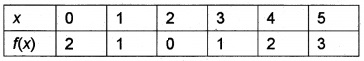2. f(x) = x2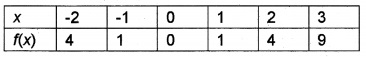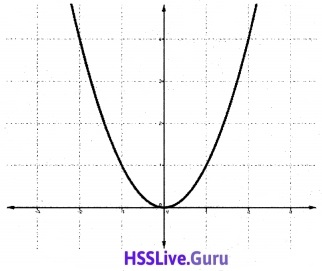3. f(x) = x3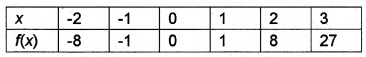4. f(x) = $$\frac{1}{x}$$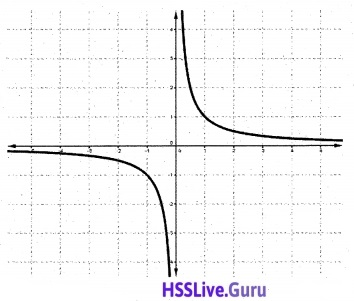5. f(x) = (x – 1)2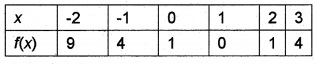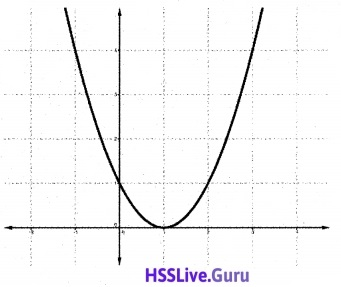6. f(x) = 3x2 – 1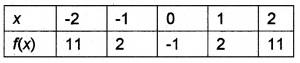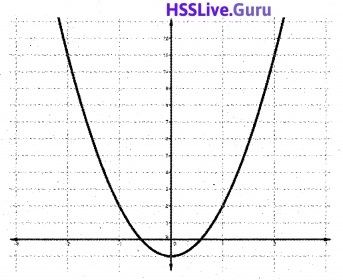7. f(x) = |x| – 2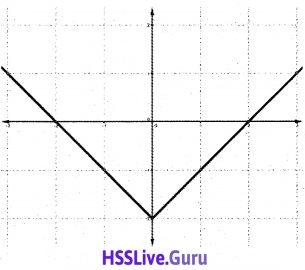Question 9.
Consider the relation, R = {(x, 2x – 1)/x ∈ A) where A = (2, -1, 3}

1. Write R in roster form. (1)
2. Write the range of R. (1)

1. x = 2 ⇒ 2x – 1 = 2(2) – 1 = 3
x = -1 ⇒ 2x – 1 = 2(-1) – 1 = -3
x = 3 ⇒ 2x – 1 = 2(3) – 1 = 5
R = {(2, 3), (-1, -3), (3, 5)}

2. Range of R = {3, -3, 5}

Question 10.
Let A = {1, 2, 3, 4, 6} and R be a relation on A defined by R = {(a, b): a, b ∈ A, b is exactly divisible by a}

1. Write R in the roster form. (1)
2. Find the domain and range of R. (1)

1. R = {(1, 1), (1, 2), (1, 3), (1, 4), (1, 6), (2, 2), (2, 4), (2, 6), (3, 3), (4, 4), (5, 5), (6, 6)}
2. Domain = {1, 2, 3, 4, 6}; Range = {1, 2, 3, 4, 6}

Question 11.
Consider the real function

1. $$f(x)=\frac{x^{2}+2 x+3}{x^{2}-8 x+12}$$
2. Find the value of x if /(x) = 1
3. Find the domain of f.

1. Given; f(x) = 1 ⇒ 1 = $$\frac{x^{2}+2 x+3}{x^{2}-8 x+12}$$
⇒ x2 – 8x + 12 = x2 + 2x + 3
⇒ 10x = 9 ⇒ x = $$\frac{9}{10}$$

2. Find the value for which denominator is zero.
⇒ x2 – 8x + 12 = 0
⇒ (x – 6)(x – 2) = 0 ⇒ x = 6, 2
Therefore domain of f is R – {2, 6).

Question 12.
If f(x) = x3 + 5x and g(x) = 2x +1, find (f + g)(2) and {fg)(1).
(f + g)(2) = f(2) + g(2) = (2)3 + 5(2) + 2(2) + 1
= 8 + 10 + 4 + 1 = 23
(fg)(1) = f(1)g(1) = (1 + 5)(2 + 1) = 6 × 3 = 18.

Question 13.
Let A = {1, 2, 3, 4, 5} and R be a relation on A defined by R = {(a, b):b = a2}

1. Write R in the roster form.
2. Find the range of R.

1. R ={(1, 1), (2, 4)}
2. Range = {1, 4}

Question 14.
Draw the graph of the function
f(x) – |x| + 1, x ∈ R